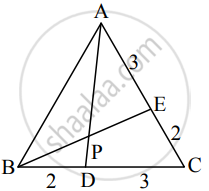# D and E divides sides BC and CA of a triangle ABC in the ratio 2 : 3 respectively. Find the position vector of the point of intersection of AD and BE and the ratio in which this point divides AD and - Mathematics and Statistics

Sum

D and E divides sides BC and CA of a triangle ABC in the ratio 2 : 3 respectively. Find the position vector of the point of intersection of AD and BE and the ratio in which this point divides AD and BE

#### SolutionLet AD and BE intersect at P.

Let A, B, C, D, E, P have position vectos bar"a", bar"b", bar"c", bar"d", bar"e", bar"p" respectively.

D and E divide segments BC and CA internally in the ratio 2 : 3.
By the section formula for internal division,

bar"d" = (2bar"c" + 3bar"b")/(2 + 3)

∴ 5bar"d" = 2bar"c" + 3bar"b"    ....(1)

and bar"e" = (2bar"a" + 3bar"c")/(2 + 3)

∴ 5bar"e" = 2bar"a" + 3bar"c"    ....(2)

From (1), 5bar"d" - 3bar"b" = 2bar"c"   ......∴ 15bar"d" - 9bar"b" = 6bar"c"

From (2), 5bar"e" - 2bar"a" = 3bar"c"   ......∴ 10bar"e" - 4bar"a" = 6bar"c"

Equating both values of 6bar"c", we get

15bar"d" - 9bar"b" = 10bar"e" - 4bar"a"

∴ 15bar"d" + 4bar"a" = 10bar"e" + 9bar"b"

∴ (15bar"d" + 4bar"a")/(15 + 4) = (10bar"e" + 9bar"b")/(10 + 9)

L.H.S is the position vector of the point which divides segment AD internally in the ratio 15 : 4

R.H.S is the position vector of the point which divides segment BE internally in the ratio 10 : 9

But P is the point of intersection of AD and BE

∴ P divides AD internally in the ratio 15 : 4 and P divides BE internally in the ratio 10 : 9

Hence, the position vector of the point of interaction of AD and BE is

bar"p" = (15bar"d" + 4bar"a")/19 = (10bar"e" + 9bar"b")/19

and it divides AD internally in the ratio 15 : 4 and BE internally in the ratio 10 : 9.

Concept: Three Dimensional (3-D) Coordinate System
Is there an error in this question or solution?